+44 (0) 203 773 6024
Get in touch with one of our tutor experts
+44 (0) 203 773 6024
GCSE

# Top Chemistry GCSE topics tutored

At MyTutor, we’ve got lots of dedicated Chemistry tutors across the UK who love helping teens achieve their best when exams come around. Since we started in 2013, we’ve given more than 250,000 one-to-one lessons, and over 1 million school pupils have used our online resource centre. Over time we’ve been able to get a strong understanding of exactly the topics in each subject that kids tend to need that extra help with.

Here are the top three Chemistry topics our students struggle with most, and some example answers which can double as handy study notes for your child to get them revision-ready.

## 1. Balance the Chemical Equation for the reaction of calcium carbonate with hydrochloric acid: CaCO3+ HCl -> CaCl2 + CO2 + H2O

To balance chemical equations we need to look at each element individually on both sides of the equation.

To start with we can add up the elements to see which are unbalanced.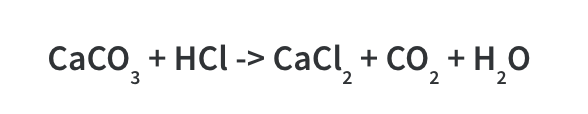There is one Calcium atom on the left and one Calcium atom on the right so this is balanced.

There is 1 Carbon atom on the left and 1 Carbon atom on the right so this is balanced.

There are 3 Oxygen atoms on the left and 3 Oxygen atoms on the right so this is balanced.

There is 1 hydrogen atom on the left but 2 on the right so this is unbalanced. Therefore we need to balance it by using 2 molecules of HCl: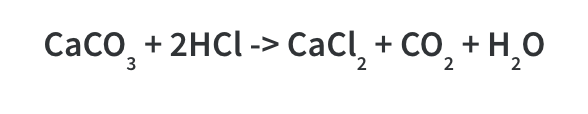Lastly, we need to check that the chlorine is balanced, and it is as we can see there are now 2 on each side. Therefore this is now a balanced equation.

Whenever we balance an equation you have to change the number of molecules used represented by the big numbers before a molecule e.g 2HCl. You cannot change the subscript (small) numbers as this is the number of each element in a molecule, and you would end up making up your own molecule that doesn’t make sense. For example you could not do H2Cl!

The easiest way to balance equations is by going through step by step and each time you make a change- check how it affects the rest of the equation.

## 2. Balance the following equation: C3H8 +O2 —-> CO2 + H2O. (There will be no need for state symbols.)

First of all, to balance these equations, list the number of each atom, for both sides of the equation. In this example, it would be:

Carbon: 3                Carbon: 1
Hydrogen: 8           Hydrogen: 2
Oxygen: 2                Oxygen: 3

The first step would be to balance out the carbons on the products side by multiplying CO2 by 3. The new equation will be

C3H8 + 02 —-> 3CO2 + H20

Now, the carbons are balanced, we will look at the hydrogens. We can multiply the number of waters on the product side by 4, to make 4 H20 molecules. This gives both sides of the equation 8 hydrogens. Next we can check our oxygens. There will be 10 oxygens on the products side and 2 on the reactants so to balance these out, we multiply the 02 on the reactants side by 5.

The final equation will be

C3H8 + 502 —-> 3CO2 + 4H20

## 3. Balance the equation for the reduction of MnO4- to Mn2+

Balancing equations is usually fairly simple. However some of them involve several steps.

You may have come across balanced equations in data booklets that look intimidating. The balanced equation for reduction of Mn7+ to Mn2+ is one such equation.

Initially one might write:

Although technically balanced (since the ox state of Mn in MnO4 is +7), this equation does not represent the full reaction that takes place which involves O molecules and H ions

The fully balanced equation is: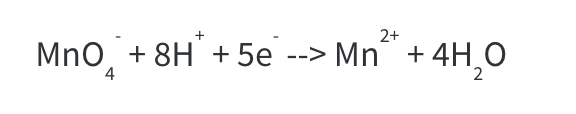To balance this, the following steps must be followed:

Step 1: Write only what’s given.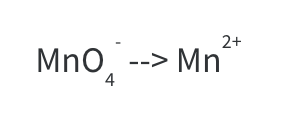Step 2: Balance all atoms except for H and O.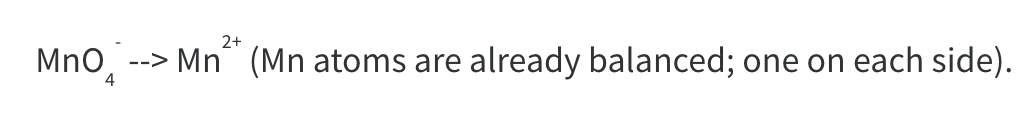Step 3: Balance Oxygen atom by adding H2O to the side where more oxygen atoms are needed. At this stage, we have 4 O atoms on the left hand side and need 4 on the RHS. One water molecule contains one O atom, so we need 4 water molecules.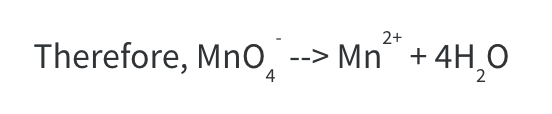Notice that now we have 4 O atoms on each side but 8 H atoms on the RHS.

Step 4: Balance H atoms by adding the required number of H+ ions to the side that is short of H atoms. At this stage we have (4 x 2) 8 H atoms on the RHS and none on the LHS.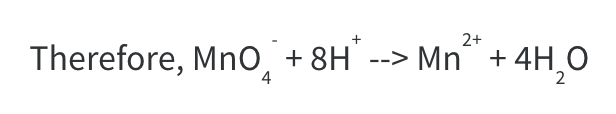Balance the charges by adding an electron, e. At this stage, the LHS has a  (-1 +8) +7 charge. The RHS has a +2 charge. If we add 5e to the LHS, the charge becomes 7 – 5 = +2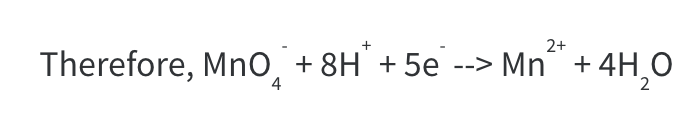By the end of step 5, we have obtained the fully balanced equation.

You can balance any equation using these steps, however, there is a slight adjustment that has to be made to step 4 sometimes.

In acidic solutions, to balance H atoms you just add H to the side lacking H atoms but in a basic solution, there is a negligible amount of H+ present. Instead, OH- is abundant. In this case, you add H2O to the side lacking H atom(s) and a OH- to the opposite side.

The net effect is that you end up adding 1 H atom to the side that lacks a H atom. If a side lacks ‘n’ number of H atoms, add ‘n’ number of H2O molecules to that side and ‘n’ number of OH- ions to  the opposite side.

## 4. Explain why chlorine (Cl2) is a gas at room temperature, but sodium chloride (NaCl) is a solid at room temperature.

The melting/boiling point of a substance determines what state of matter it takes at a certain temperature.

In Cl2 there are covalent bonds between the atoms forming simple molecules. There are weak attractions between molecules of Cl2 meaning little energy is needed to break these forces of attraction and therefore Cl2 has a low boiling point.

NaCl on the other hand has strong electrostatic forces of attraction between the positively charged Na ion and the negatively charged Cl ion. NaCl forms a giant ionic lattice that requires high amounts of energy to break and therefore has a high melting point.

## 5. What is the difference between ionic, covalent and metallic bonding?

Ionic bonding occurs when transfer of electrons takes place. One atom (or molecule) donates one or more electrons to another. Since electrons have a negative charge, this leaves the donating atom electron deficient (+ve charged ion) and the other atom electron rich ( -ve charged ion). The ions then attract each other through electrostatic forces of attraction as they are oppositely charged.

Covalent bonding occurs when atoms/molecules share pairs of electrons.

Metallic bonding is bonding that occurs in metals. This leads to giant structures of metal atoms arranged in a regular pattern. The outer shell electrons of metals are delocalised (free to move around) and so a metallic structure is a regular arrangement of +ve charged ions with negative electrons in between, held together by electrostatic forces of interaction.

8 years ago
A Level

### MyTutor: A new concept in affordable, convenient tutoring

This is from Student Guide Students put tutor hats on to earn money Students are being... Read more

8 years ago